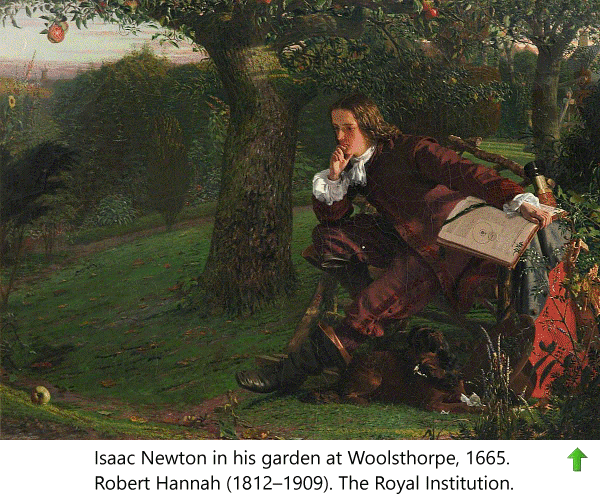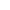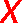Sir Isaac Newton (1643-1727) English mathematician and physicist.Newton's Three Laws of Motion.

Newton's First Law of Motion States: An object in motion will remain in motion unless an external force acts upon it. Similarly, if an object is at rest, it will remain at rest unless an external force acts upon it.

Newton's Second Law of Motion States: When a force acts upon an object it will cause the object to accelerate.

Newton's Third Law of Motion States: For every action there is an equal and opposite reaction.Close and return to previous page